# 21 Resources for Learning Math for Data Science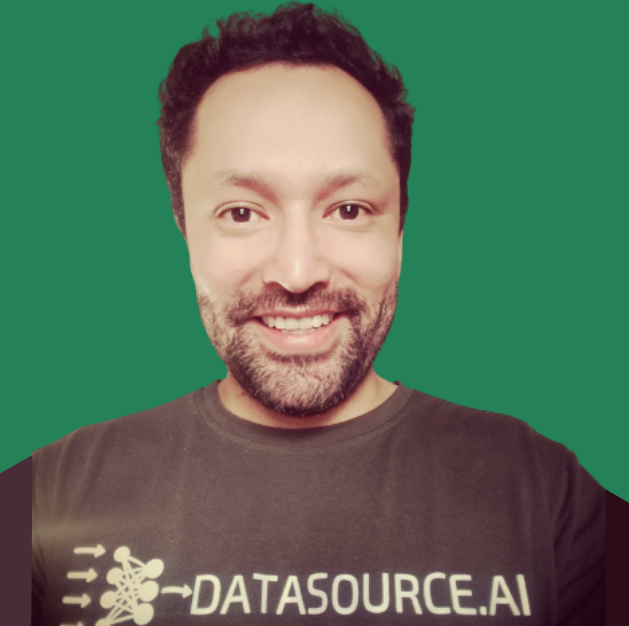Jan 19, 2021

This is probably one of the biggest worries of those starting in the area of data science, learning/refreshing mathImage by DataSource.ai

Let’s be honest, most people didn’t do very well in math in school, maybe not even in college, and this is very scary and creates a barrier for those who want to explore this discipline called data science.

A few days ago I published a post in Towards Data Scienceand right here on our blog called Study Plan for Learning Data Science Over the Next 12 Months, where I gave some quarterly recommendations and made an emphasis on studying mathematics and statistics for this first quarter, and from which I received many questions about exactly which materials I recommended. Well, this post answers those questions. But before that, I want to give you a context.

Leaving aside the factors or reasons that have led most people to hate math, it is a reality that we need it in data science. For me, one of the biggest shortcomings I found in mathematics was its lack of applicability in the real world, I didn’t see a reason for intermediate and advanced mathematics, such as multivariate calculus. I confess that in school and college I didn’t like them for that reason, but I always did well and got good scores and averages above the majority (especially in statistics). But I still didn’t see how I could use a derivative or a matrix in the real world. I finally ended up as a software engineer and once I entered the world of data science I was able to make the connection between mathematics, statistics, and the real world.

On the other hand, it is important to clarify that we do not need a master’s degree in pure mathematics to do data science projects. As I mentioned in previous posts there is a big debate in the community about how much math we need to do a good job as data scientists.

We could say that data science is divided into two major fields of work: research and production

By research, we mean the part of research and development, which normally takes place within a large company (usually a tech company), or which has focused on cutting-edge technological issues (such as medical research). Or it is also an area that is developed within universities. This sector has very limited job offers.

• The great advantage is the deep knowledge of algorithms and their implementations, as well as being a person capable of creating variations of existing algorithms, to improve them. Or even create new machine learning algorithms.
• The disadvantage is the unpractical nature of their work. It is a very theoretical work, in which often the only objective is to publish papers and is far from the business use cases in general. For reference on this, I recently read this post on Reddit, I recommend you read it.
By production, we refer to the practical side of this discipline, where you’ll use generally and in your day to day job libraries such as scikit-learn, Tensorflow, Keras, Pytorch, and others. These libraries operate like a black box, where you enter data, you get an output, but you don’t know in detail what happened in the process. This also has its advantages and disadvantages, but it certainly makes life much easier when putting useful models into production. What I don’t recommend is to use them blindly, where you don’t have the minimum bases of mathematics to understand a little of their fundamentals and that is the objective of this post, to guide you and recommend you some valuable resources to have the necessary bases and not to operate blindly those libraries.

So if you decide to focus on Research and Development, you are going to need mathematics and statistics in depth (very in-depth). If you are going to go for the practical part, the libraries will help you deal with most of it, under the hood. It should be noted that most job offers are in the practical side.

Well, after the previous remarks, it is time to define which are the specific topics needed to have an initial basis in mathematics for data science.

• Linear Algebra: This subject is important to have the fundamentals of working with data in vector and matrix form, to acquire skills to solve systems of linear algebraic equations, and to find the basic matrix decompositions and the general understanding of their applicability.
• Calculus: Here it is important to study functional maps, limits (in case of sequences, functions of one and several variables), differentiation (from a single variable to multiple cases), integration, thus sequentially building a foundation for basic optimization. It is also important here to study gradient descent.
• Probability theory: Here you should learn about random variables, i.e. a variable whose values are determined by a random experiment. Random variables are used as a model for the data generation processes we want to study. The properties of the data are deeply linked to the corresponding properties of the random variables, such as expected value, variance, and correlations.
Note: these subjects are much deeper than what I just mentioned, this is simply a guide of the subjects and resources recommended to approach mathematics in the field of data science.

Now that we have a better idea of the path we should take, let’s examine the recommended resources to address this topic. We will divide them into basic, intermediate, and advanced. In the advanced ones, we’ll have resources focused on deep learning

Basics: in this first section of resources we’ll recommend the mathematical basics. Mathematical thinking, algebra, and how to implement math with python.

# 1- Introduction to mathematical thinking

Price: Free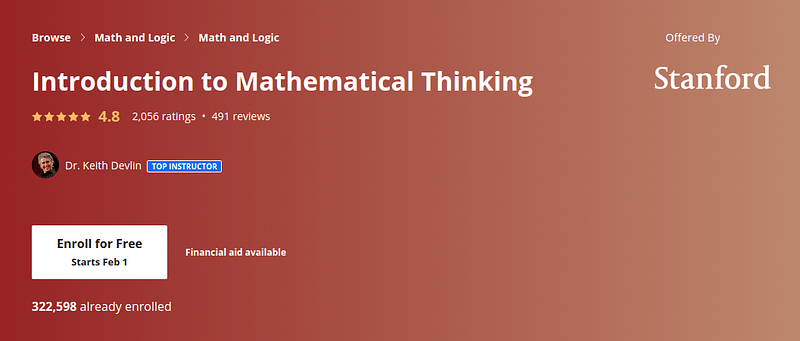Image by Coursera

Description: Learn how to think the way mathematicians do — a powerful cognitive process developed over thousands of years.

Mathematical thinking is not the same as doing mathematics — at least not as mathematics is typically presented in our school system. School math typically focuses on learning procedures to solve highly stereotyped problems. Professional mathematicians think a certain way to solve real problems, problems that can arise from the everyday world, or from science, or from within mathematics itself. The key to success in school math is to learn to think inside-the-box. In contrast, a key feature of mathematical thinking is thinking outside-the-box — a valuable ability in today’s world. This course helps to develop that crucial way of thinking.

# 2- Mathematical Foundation for AI and Machine Learning

Price: \$46.99 usd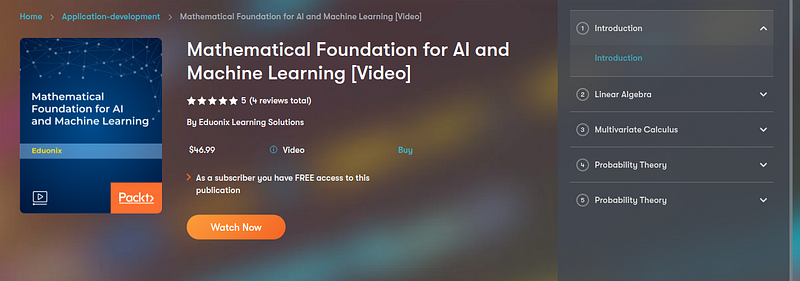Image by Packt

Description: Artificial Intelligence has gained importance in the last decade with a lot depending on the development and integration of AI in our daily lives. The progress that AI has already made is astounding with innovations like self-driving cars, medical diagnosis and even beating humans at strategy games like Go and Chess. The future for AI is extremely promising and it isn’t far from when we have our own robotic companions. This has pushed a lot of developers to start writing codes and start developing for AI and ML programs. However, learning to write algorithms for AI and ML isn’t easy and requires extensive programming and mathematical knowledge. Mathematics plays an important role as it builds the foundation for programming for these two streams. And in this course, we’ve covered exactly that. We designed a complete course to help you master the mathematical foundation required for writing programs and algorithms for AI and ML.

# 3- Math for Programmers

Price: \$47.99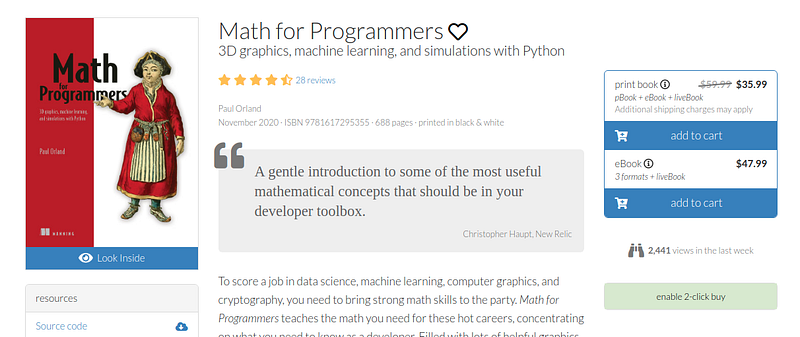Image by Manning

Description: In Math for Programmers you’ll explore important mathematical concepts through hands-on coding. Filled with graphics and more than 300 exercises and mini-projects, this book unlocks the door to interesting–and lucrative!–careers in some of today’s hottest fields. As you tackle the basics of linear algebra, calculus, and machine learning, you’ll master the key Python libraries used to turn them into real-world software applications.

# 4- Algebra 1

Price: Free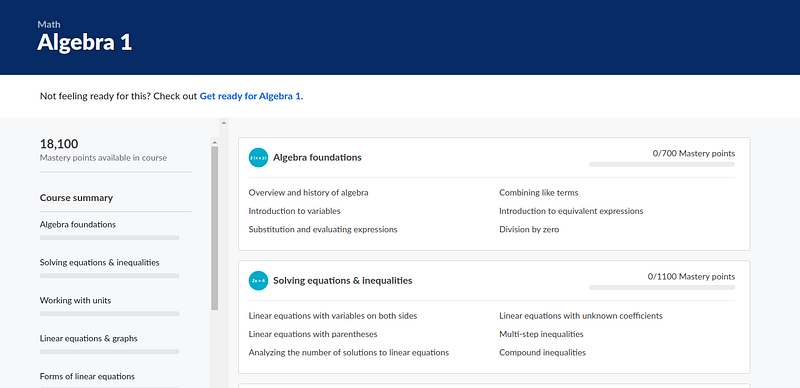Image by Khanacademy

# 5- Algebra 2

Price: Free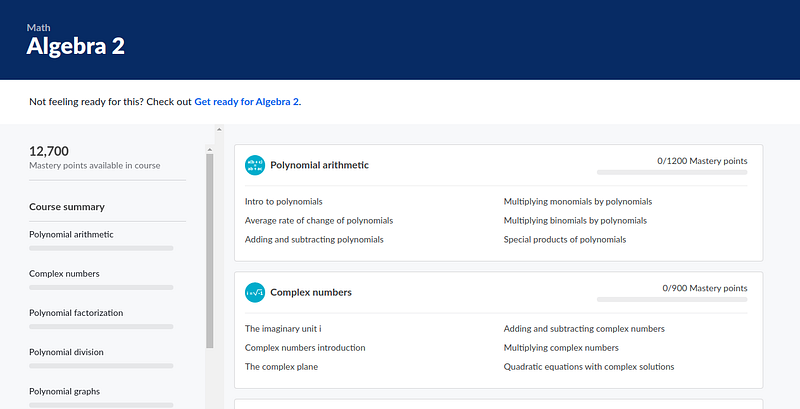Image by Khanacademy

# 6- Master Math by Coding in Python

Price: \$12.99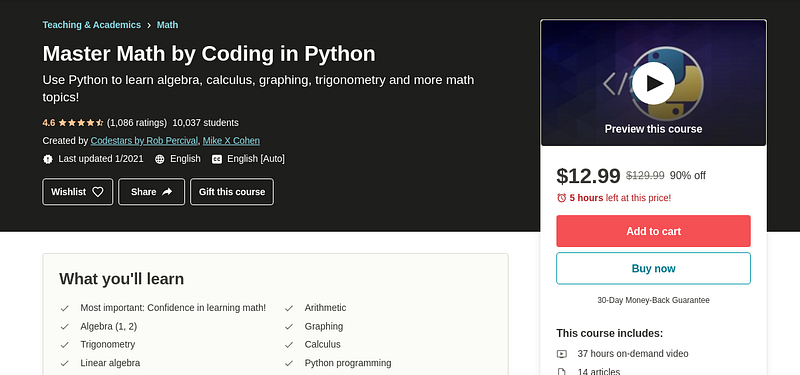Image by Udemy

Description: You can learn a lot of math with a bit of coding!

Many people don’t know that Python is a really powerful tool for learning math. Sure, you can use Python as a simple calculator, but did you know that Python can help you learn more advanced topics in algebra, calculus, and matrix analysis? That’s exactly what you’ll learn in this course.

Let me guess what you are thinking:

• “But I don’t know Python!”That’s okay! This course is aimed at complete beginners; I take you through every step of the code. You don’t need to know anything about Python, although it’s useful if you already have some programming experience.
• “But I’m not good at math!”You will be amazed at how much better you can learn math by using Python as a tool to help with your courses or your independent study. And that’s exactly the point of this course: Python programming as a tool to learn mathematics. This course is designed to be the perfect addition to any other math course or textbook that you are going through.

# 7- Introduction to Linear Models and Matrix Algebra

Price: Free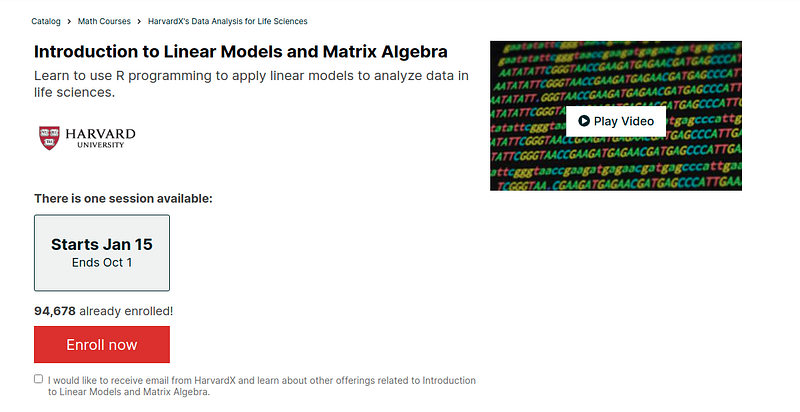Image by Edx

Description: Matrix Algebra underlies many of the current tools for experimental design and the analysis of high-dimensional data. In this introductory online course in data analysis, we will use matrix algebra to represent the linear models that commonly used to model differences between experimental units. We perform statistical inference on these differences. Throughout the course we will use the R programming language to perform matrix operations.

Given the diversity in educational background of our students we have divided the series into seven parts. You can take the entire series or individual courses that interest you. If you are a statistician you should consider skipping the first two or three courses, similarly, if you are biologists you should consider skipping some of the introductory biology lectures. Note that the statistics and programming aspects of the class ramp up in difficulty relatively quickly across the first three courses. You will need to know some basic stats for this course. By the third course will be teaching advanced statistical concepts such as hierarchical models and by the fourth advanced software engineering skills, such as parallel computing and reproducible research concepts.

# 8- Applying Math with Python

Price: \$20.99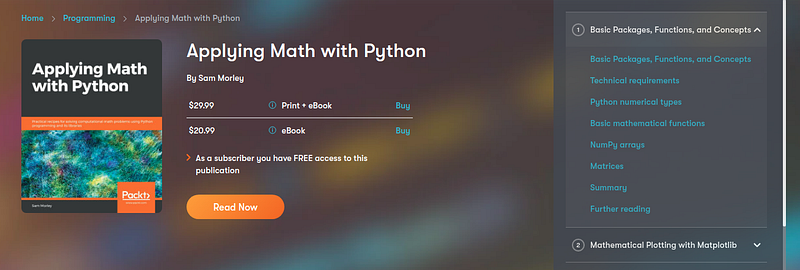Image by Packt

Description: Python, one of the world’s most popular programming languages, has a number of powerful packages to help you tackle complex mathematical problems in a simple and efficient way. These core capabilities help programmers pave the way for building exciting applications in various domains, such as machine learning and data science, using knowledge in the computational mathematics domain.

The book teaches you how to solve problems faced in a wide variety of mathematical fields, including calculus, probability, statistics and data science, graph theory, optimization, and geometry. You’ll start by developing core skills and learning about packages covered in Python’s scientific stack, including NumPy, SciPy, and Matplotlib. As you advance, you’ll get to grips with more advanced topics of calculus, probability, and networks (graph theory). After you gain a solid understanding of these topics, you’ll discover Python’s applications in data science and statistics, forecasting, geometry, and optimization. The final chapters will take you through a collection of miscellaneous problems, including working with specific data formats and accelerating code.

By the end of this book, you’ll have an arsenal of practical coding solutions that can be used and modified to solve a wide range of practical problems in computational mathematics and data science.

Intermediate: in this second section we will recommend resources focused on calculation and probability.

# 9- Calculus 1

Price: Free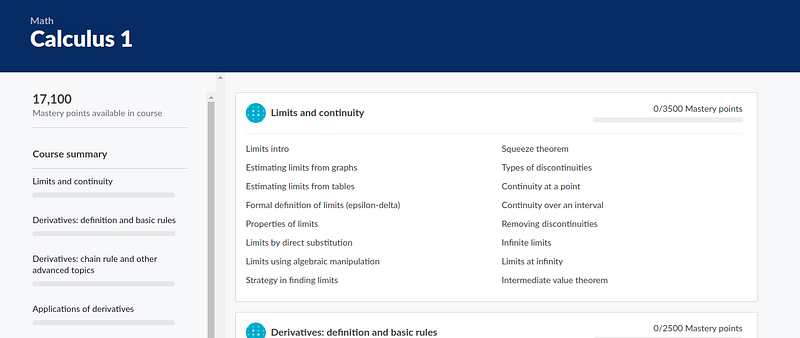Image by Khanacademy

# 10- Calculus 2

Price: Free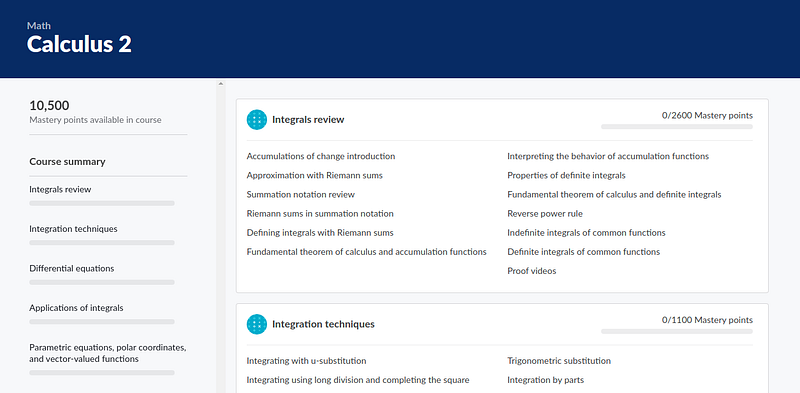Image by Khanacademy

# 11- Multivariable calculus

Price: Free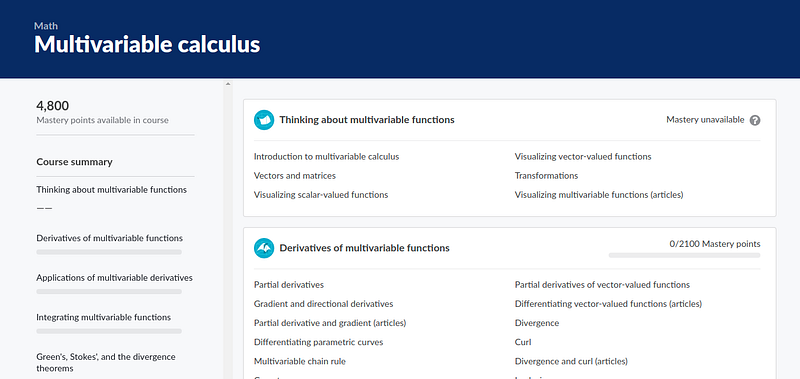Image by Khanacademy

# 12- Mathematics for Data Science Specialization

Price: Free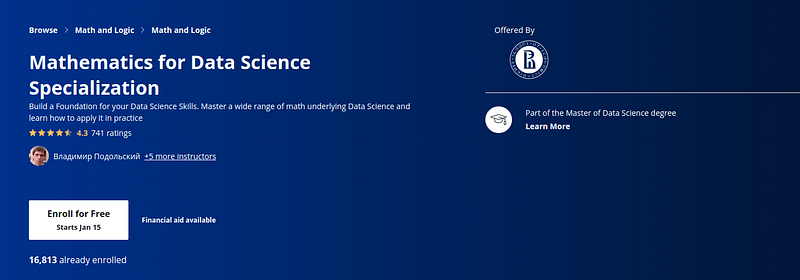Image by Coursera

Description: Behind numerous standard models and constructions in Data Science there is mathematics that makes things work. It is important to understand it to be successful in Data Science. In this specialisation we will cover wide range of mathematical tools and see how they arise in Data Science. We will cover such crucial fields as Discrete Mathematics, Calculus, Linear Algebra and Probability. To make your experience more practical we accompany mathematics with examples and problems arising in Data Science and show how to solve them in Python.

Each course of the specialisation ends with a project that gives an opportunity to see how the material of the course is used in Data Science. Each project is directed at solving practical problem in Data Science. In particular, in your projects you will analyse social graphs, predict estate prices and uncover hidden relations in the data.

# 13- Practical Discrete Mathematics

Price: \$24.99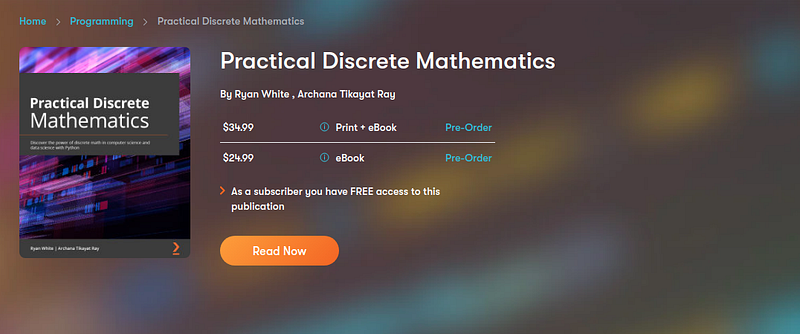Image by Packt

Description: Discrete mathematics is a field of math that deals with studying finite and distinct elements. The theories and principles of discrete math are widely used in solving complexities and building algorithms in computer science and computing data in data science. It helps you to understand algorithms, binary, and general mathematics that is commonly used in data-driven tasks.

Learn Discrete Mathematics is a comprehensive introduction for those who are new to the mathematics of countable objects. This book will help you get up-to-speed with implementing discrete math principles to take your programming skills to another level. You’ll learn the discrete math language and methods crucial to studying and describing objects and functions in branches of computer science and machine learning. Complete with real-world examples, the book covers the internal workings of memory and CPUs, analyzes data for useful patterns, and shows you how to solve problems in network routing, encryption, and data science.

By the end of this book, you’ll have a deeper understanding of discrete mathematics and its applications in computer science, and get ready to work on real-world algorithm development and machine learning.

# 14- Math for Data Science and Machine Learning: University Level

Price: \$12.99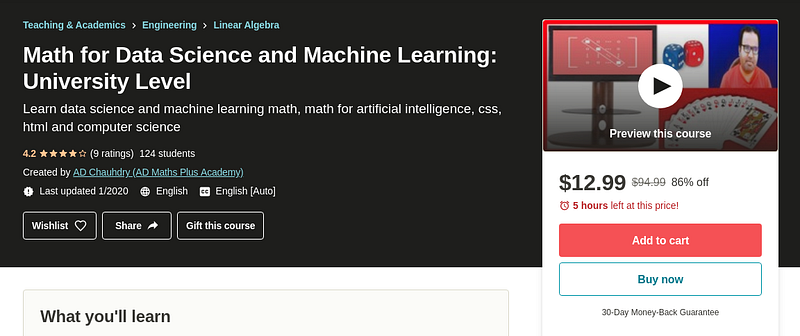Image by Udemy

Description: In this course we will learn math for data science and machine learning. We will also discuss the importance of Math for data science and machine learning in practical word. Moreover, Math for data science and machine learning course is bundle of two courses of linear algebra and probability and statistics. So, students will learn complete contents of probability and statistics and linear algebra. It is not like that you will not complete all the contents in this 7 hours videos course. This is a beautiful course and I have designed this course according to the need of the students.

Linear algebra and probability and statistics is usually offered for the students of data science, machine learning, python and IT students. So, that’s why I have prepared this dual course for different sciences.

I have taught this course multiple times on my universities classes. It is offered usually in two different modes like, it is offered as linear algebra for 100 marks paper and probability and statistics as another 100 marks paper for two different or in a same semesters. I usually focus on the method and examples while teaching this course. Examples clear the concepts of the students in a variety of way like, they can understand the main idea that instructor want to deliver if they feel typical the method of the subject or topics. So, focusing on example makes the course easy and understandable for the students.

# 15- Data Science Math Skills

Price: Free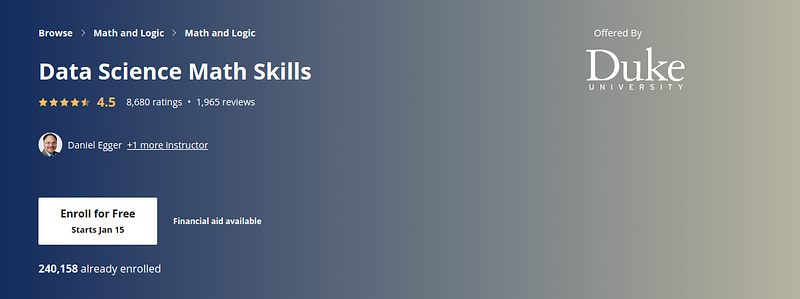Image by Coursera

Description: Data science courses contain math — no avoiding that! This course is designed to teach learners the basic math you will need in order to be successful in almost any data science math course and was created for learners who have basic math skills but may not have taken algebra or pre-calculus. Data Science Math Skills introduces the core math that data science is built upon, with no extra complexity, introducing unfamiliar ideas and math symbols one-at-a-time.

Learners who complete this course will master the vocabulary, notation, concepts, and algebra rules that all data scientists must know before moving on to more advanced material.

Advanced: in this last section we will focus on the statistical part (probability theory) and the application of mathematics to deep learning algorithms.

# 16- Statistics and probability

Price: Free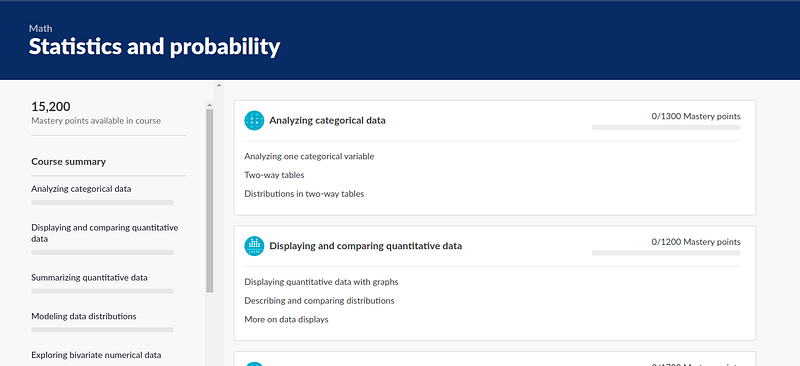Image by Khanacademy

# 17- Intro to Inferential Statistics

Price: Free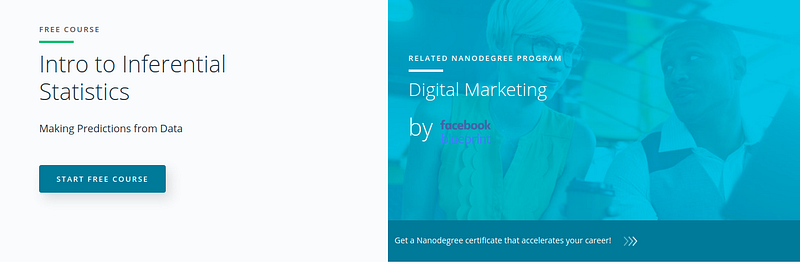Image by Udacity

Description: Inferential statistics allows us to draw conclusions from data that might not be immediately obvious. This course focuses on enhancing your ability to develop hypotheses and use common tests such as t-tests, ANOVA tests, and regression to validate your claims.

# 18- Statistical Methods and Applied Mathematics in Data Science

Price: \$124.99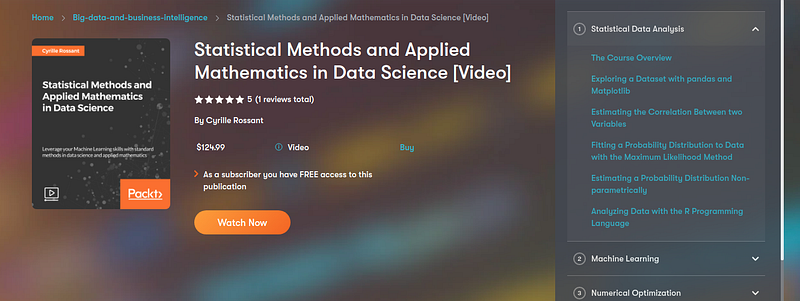Image by Packt

Description: Machine learning and data analysis are the center of attraction for many engineers and scientists. The reason is quite obvious: its vast application in numerous fields and booming career options. And Python is one of the leading open source platforms for data science and numerical computing. IPython, and its associated Jupyter Notebook, provide Python with efficient interfaces to for data analysis and interactive visualization, and they constitute an ideal gateway to the platform. If you are among those seeking to enhance their capabilities in machine learning, then this course is the right choice.

Statistical Methods and Applied Mathematics in Data Science provides many easy-to-follow, ready-to-use, and focused recipes for data analysis and scientific computing. This course tackles data science, statistics, machine learning, signal and image processing, dynamical systems, and pure and applied mathematics. You will apply state-of-the-art methods to various real-world examples, illustrating topics in applied mathematics, scientific modeling, and machine learning. In short, you will be well versed with the standard methods in data science and mathematical modeling.

# 19- Exploring Math for Programmers and Data Scientists

Price: Free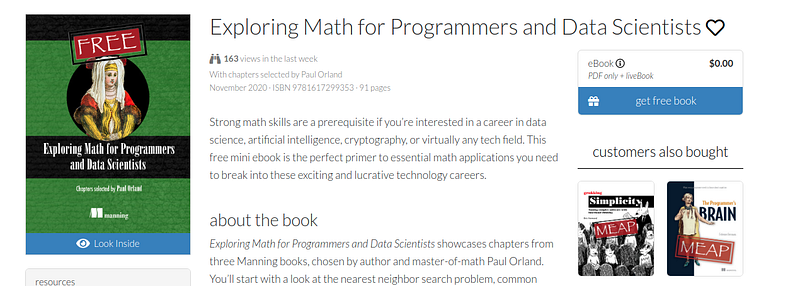Image by Manning

Description: Exploring Math for Programmers and Data Scientists showcases chapters from three Manning books, chosen by author and master-of-math Paul Orland. You’ll start with a look at the nearest neighbor search problem, common with multidimensional data, and walk through a real-world solution for tackling it. Next, you’ll delve into a set of methods and techniques integral to Principal Component Analysis (PCA), an underlying technique in Latent Semantic Analysis (LSA) for document retrieval. In the last chapter, you’ll work with digital audio data, using mathematical functions in different and interesting ways. Begin sharpening your competitive edge with the fun and fascinating math in this (free!) practical guide!

# 20- Hands-On Mathematics for Deep Learning

Price: \$27.99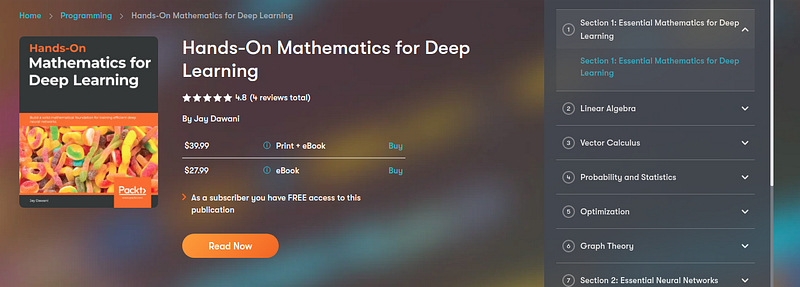Image by Packt

Description: Most programmers and data scientists struggle with mathematics, having either overlooked or forgotten core mathematical concepts. This book uses Python libraries to help you understand the math required to build deep learning (DL) models.

You’ll begin by learning about core mathematical and modern computational techniques used to design and implement DL algorithms. This book will cover essential topics, such as linear algebra, eigenvalues and eigenvectors, the singular value decomposition concept, and gradient algorithms, to help you understand how to train deep neural networks. Later chapters focus on important neural networks, such as the linear neural network and multilayer perceptrons, with a primary focus on helping you learn how each model works. As you advance, you will delve into the math used for regularization, multi-layered DL, forward propagation, optimization, and backpropagation techniques to understand what it takes to build full-fledged DL models. Finally, you’ll explore CNN, recurrent neural network (RNN), and GAN models and their application.

By the end of this book, you’ll have built a strong foundation in neural networks and DL mathematical concepts, which will help you to confidently research and build custom models in DL.

# 21- Math and Architectures of Deep Learning

Price: \$39.99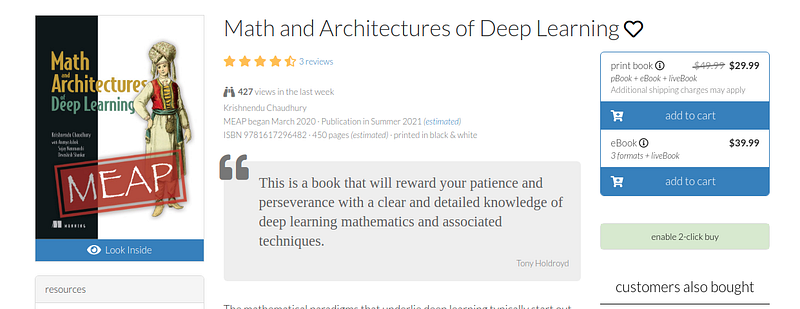Image by Manning

Description: Math and Architectures of Deep Learning sets out the foundations of DL in a way that’s both useful and accessible to working practitioners. Each chapter explores a new fundamental DL concept or architectural pattern, explaining the underpinning mathematics and demonstrating how they work in practice with well-annotated Python code. You’ll start with a primer of basic algebra, calculus, and statistics, working your way up to state-of-the-art DL paradigms taken from the latest research. By the time you’re done, you’ll have a combined theoretical insight and practical skills to identify and implement DL architecture for almost any real-world challenge.

Conclusion

This is an extensive recommendation on resources for learning mathematics for data science, following the previous post about the path to follow in this year 2021 to learn data science

When we have limited time for study, we should select those that we feel best and those that fit our style. For example, you might prefer videos about books, so go ahead and choose what suits you best. This material is sufficient whether you want to take a brief look at the mathematics, or if you want to go deeper into it. I hope you find it useful.

If you have other recommendations for courses, books or videos, please leave them in the comments so that we can all create links of interest.

Note: we are building a private community in Slack of data scientist, if you want to join us you can register here: https://www.datasource.ai/en#slack

“21 Resources for Learning Math for Data Science”
– Daniel Morales

### Related Stories

Nov 25, 2021

#### 5 Tips To Ace Your Job Interview For A Data Scientist Opening

5 Tips To Ace Your Job Interview For A Data Scientist Opening.PNG 795.94 KBImage SourceAspiring data scientists have a bright future ahead of them....By Daniel Morales
Nov 12, 2021

#### When to Avoid Deep LearningBy Matt Przybyla
Oct 16, 2021

#### 6 Advanced Statistical Concepts in Data Science

The article contains some of the most commonly used advanced statistical concepts along with their Python implementation.In my previous articles Be...By Nagesh Singh Chauhan